## Cross Reference Down

#### Clenshaw, CV, Olver, FWJ and Turner, PR (1989). Level-Index Arithmetic - An Introductory Survey. Lecture Notes in Mathematics 1397, pp. 95-168.

This work cites the following items of the Benford Online Bibliography:

 Barlow, JL and Bareiss, EH (1985). On Roundoff Error Distributions in Floating Point and Logarithmic Arithmetic. Computing 34(4), pp. 325-347. ISSN/ISBN:0010-485X. DOI:10.1007/BF02251833.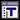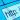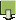Benford, F (1938). The law of anomalous numbers. Proceedings of the American Philosophical Society, Vol. 78, No. 4 (Mar. 31, 1938), pp. 551-572.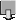Feldstein, A and Turner, P (1986). Overflow, Underflow, and Severe Loss of Significance in Floating-Point Addition and Subtraction. IMA Journal of Numerical Analysis 6, pp. 241-251. DOI:10.1093/imanum/6.2.241.Flehinger, BJ (1966). On the Probability that a Random Integer has Initial Digit A. American Mathematical Monthly 73(10), pp. 1056-1061. ISSN/ISBN:0002-9890. DOI:10.2307/2314636.Goodman, RH, Feldstein, A and Bustoz, J (1985). Relative Error in Floating-Point Multiplication. Computing 35, pp. 127-139. ISSN/ISBN:1436-5057. DOI:10.1007/BF02260500.Hamming, R (1970). On the distribution of numbers. Bell Syst. Tech. J. 49(8), pp. 1609-1625. ISSN/ISBN:0005-8580. DOI:10.1002/j.1538-7305.1970.tb04281.x.Matula, VV and Kornerup, P (1980). Foundations of Finite Precision Rational Arithmetic. pp 85-111 in: Alefeld, G, Grigorieff, RD (eds.) Fundamentals of Numerical Computation (Computer-Oriented Numerical Analysis), Computing Supplementum 2, Springer, Wien-New York.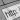Olver, FWJ (1982). Further Developments of Rp and Ap Error Analysis. IMA Journal of Numerical Analysis 2(3), pp. 249-274. ISSN/ISBN:0272-4979. DOI:10.1093/imanum/2.3.249.Pinkham, RS (1961). On the Distribution of First Significant Digits. Annals of Mathematical Statistics 32(4), pp. 1223-1230. ISSN/ISBN:0003-4851.Raimi, RA (1969). On Distribution of First Significant Figures. American Mathematical Monthly 76(4), pp. 342-348. ISSN/ISBN:0002-9890. DOI:10.2307/2316424.Turner, PR (1982). The Distribution of Leading Significant Digits. IMA Journal orf Numerical Analysis 2(4), 407-412. ISSN/ISBN:0272-4979. DOI:10.1093/imanum/2.4.407.Turner, PR (1984). Further Revelations on L.S.D.. IMA Journal of Numerical Analysis 4(2), 225-231. ISSN/ISBN:0272-4979. DOI:10.1093/imanum/4.2.225.